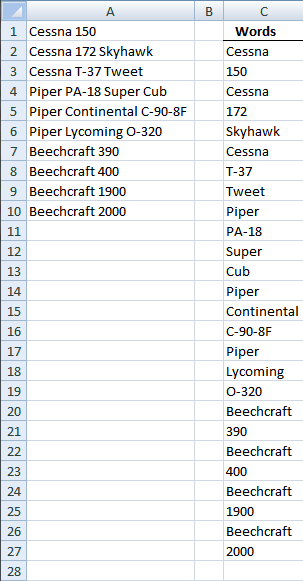Author: Oscar Cronquist Article last updated on December 30, 2018

This post describes how to split words in a cell range into a cell each using a custom function. I hope this picture explains it all:The cell range is A1:A10 and contain words.

### The array formula in C2:C27 contains an udf:

1. Select all the cells to be filled, C2:C27
2. Type the array formula SplitWords(\$A\$1:\$A\$10) into the formula bar.

### How to create an array formula

1. Copy (Ctrl + c) and paste (Ctrl + v) array formula into formula bar.
2. Press and hold Ctrl + Shift.
3. Press Enter once.
4. Release all keys.

User defined function: Read Rick Rothstein's (MVP - Excel) comment!

```
Function SplitWords(rng As Range) As Variant()

Dim x As Variant, Wrds() As Variant, Cells_row As Long
Dim Cells_col As Long, Words As Long, y() As Variant
ReDim y(0)
Wrds = rng.Value
For Cells_row = LBound(Wrds, 1) To UBound(Wrds, 1)
For Cells_col = LBound(Wrds, 2) To UBound(Wrds, 2)
x = Split(Wrds(Cells_row, Cells_col))
For Words = LBound(x) To UBound(x)
y(UBound(y)) = x(Words)
ReDim Preserve y(UBound(y) + 1)
Next Words
Next Cells_col
Next Cells_row

SplitWords = Application.Transpose(y)

End Function

```

### Where to copy the code

1. Click "Developer" tab on the ribbon
How to enable developer tab on the ribbon
2. Click "Visual Basic" button
3. Insert a new module
4. Copy this udf example and paste it into new module

### Download Excel fileextract-words-from-a-range-udf.xls